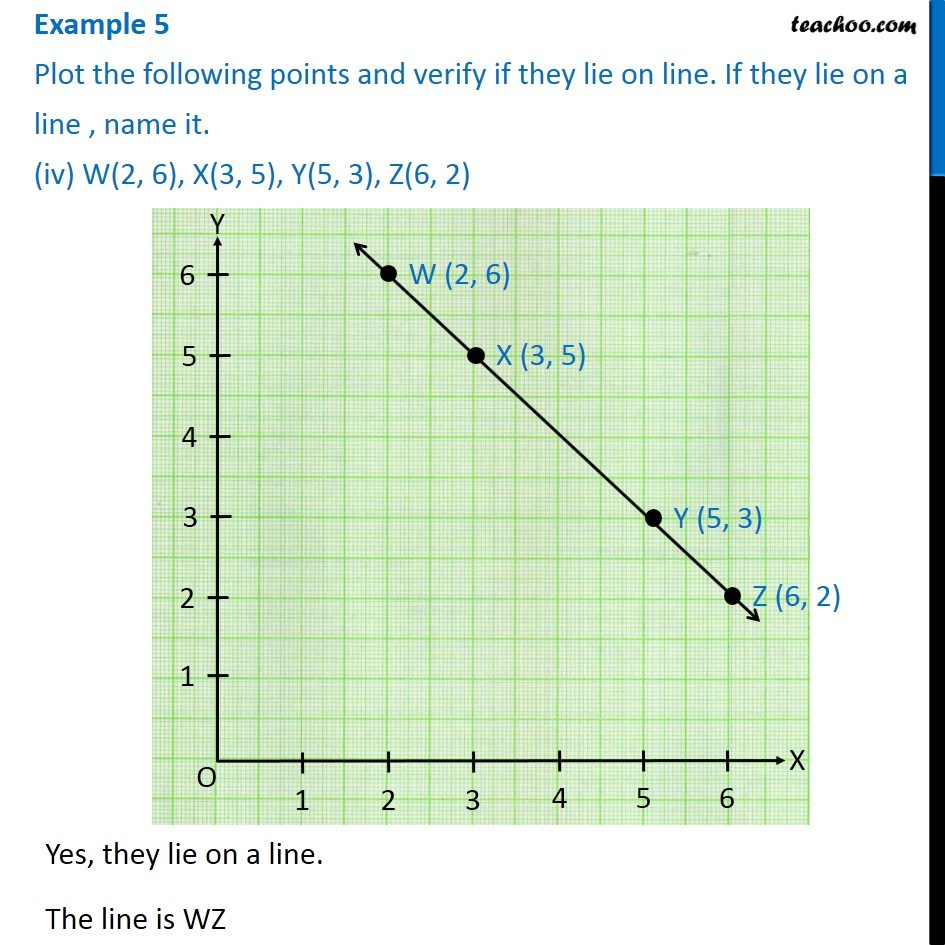1. Chapter 15 Class 8 Introduction to Graphs
2. Serial order wise
3. Examples

Transcript

Example 5 Plot the following points and verify if they lie on line. If they lie on a line , name it. (iv) W(2, 6), X(3, 5), Y(5, 3), Z(6, 2)Yes, they lie on a line. The line is WZ